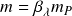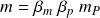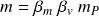# Mass

The New Foundation Model distinguishes between rest mass and inertial mass in elementary particles. Inertial mass is a variable quantity of mass determined by a particle’s wavelength, while rest mass is a fixed quantity of mass for a given particle type. The mass formula works for both types of mass.

## Standard formula: Inertial mass

Inertial mass is inversely proportional to a particle’s wavelength, so the standard formula applies the wavelength operator to the Planck massInertial mass is a measure of a particle’s spatial distribution in unit dimension M. It is one of the three correlated unit dimensions making up the first part of the 2-part energy mechanism.

Applying the wavelength operator in the mass unit dimension transforms the maximum Planck mass potential into an observable quantity of inertial mass.

### Observable

The standard form of the mass formula is created using any of three equivalent forms of the wavelength operator in each of the fundamental unit dimensions.

## Expanded formula: Inertial mass

The mass formula also uses the expanded form of the wavelength operator to find inertial mass. The expanded wavelength operator is the product of the rest mass and momentum operators.

Substituting the expanded operators produces the same transformations in the mass unit dimension.

Replacing the wavelength operator with rest mass and momentum operators gives

### Observable

The expanded form of the mass formula applies the two operators to the Planck mass potentialThe expanded form of the mass formula uses three equivalent forms of the rest mass and momentum operators multiplied by the Planck mass to produce a particle’s inertial mass.

Because the momentum and velocity operators are equal, additional forms of the mass formula can be created by substituting the velocity operator for the momentum operatorThis form of the equation produces the right answer but gives the wrong physical description of the particle or system. Applying these two operators to the maximum Planck potential gives the inertial mass observable .